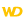• Coordinates
• Drawing in PHP
• Drawing in Browser code
WINDEVWEBDEVWINDEV MobileOthers<Image variable>.DrawPoint (Function)
In french: <Variable Image>.DessinePoint
Draws a point in a variable of type Image.Caution: A specific configuration is required to use this function in Linux. For more details, see The drawings.
Example
MyImage is Image = "Test.gif"
// Draw a light yellow point in an Image variable
MyImage.DrawPoint(10, 50, LightYellow)
IMG_MyDrawing = MyImage
Syntax

Drawing a point by specifying its coordinates

<Image variable>.DrawPoint(<X-coordinate> , <Y-coordinate> [, <Point color> [, <Opacity>]])
<Image variable>: Image variable
Name of the Image variable to be used.
<X-coordinate>: Integer
Horizontal position (in pixels) of the point to draw.
<Y-coordinate>: Integer
Vertical position (in pixels) of the point to draw.
<Point color>: Integer or constant (optional)
Color that must be assigned to the point. This color can correspond to:
If this parameter is not specified, the color of the point:
<Opacity>: Optional integer
Opacity of color, included between 0 (transparent) and 255 (opaque). An opacity sets to 128 indicates that the relevant pixel will have a transparency of 50% when it is drawn on the screen or saved in an image in PNG format.
Remark: This opacity will be taken into account if the drawing found in the image was initialized by the dWithOpacity constant (<Image variable>.StartDrawing).Drawing a point via a Point variable

<Image variable>.DrawPoint(<Point> [, <Point color> [, <Opacity>]])
<Image variable>: Image variable
Name of the Image variable to be used.
<Point>: Point variable
Name of the Point variable containing the coordinates of the point to draw.
<Point color>: Integer or constant (optional)
Color that must be assigned to the point. This color can correspond to:
If this parameter is not specified, the color of the point:
<Opacity>: Optional integer
Opacity of color, included between 0 (transparent) and 255 (opaque). An opacity sets to 128 indicates that the relevant pixel will have a transparency of 50% when it is drawn on the screen or saved in an image in PNG format.
Remark: This opacity will be taken into account if the drawing found in the image was initialized by the dWithOpacity constant (<Image variable>.StartDrawing).
Remarks

Coordinates

Coordinates are specified with respect to the upper-left corner of the image (coordinates: (0.0)).
Related Examples:Unit examples (WINDEV): The drawing functions [ + ] Using the main drawing functions of WINDEV to:- Initialize an Image control for drawing- Draw simple shapes- Write a text into a drawing- Change the color in a drawing
Business / UI classification: Neutral code
Component: wd280pnt.dll
Minimum version required
• Version 24